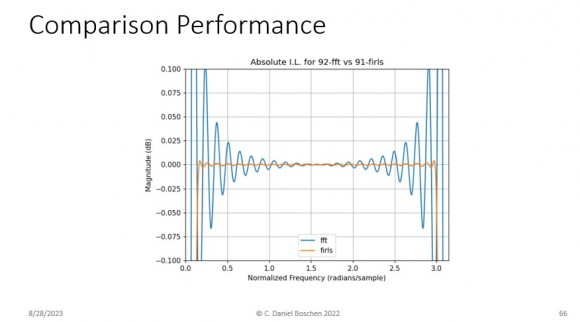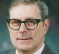## Stereo to complex sampleStarted by 4 weeks ago5 replieslatest reply 4 weeks ago177 views

A fast fourier transform works in complex numbers, and each sine or cosine wave is a composition of two complex waves. For instance the two complex waves (1, i, -1, -i, …) and (1, -i, -1, i, …) averaging to form cosine (1, 0, -1, 0, …)

Complex numbers are formed by real part and imaginary part. And stereo sample is formed by left channel and right channel. So couldn’t a stereo sound be in one FFT by mapping stereo samples to complex samples?

Real=Left, Imaginary=Right, would be one of the direct assignments of stereo sound to complex sound, the other being Real=Right, Imaginary=Left.

Real=(Left+Right)÷2, Imaginary=(Left-Right)÷2, would be more compatible with mono sound being real sound.

Is there a standardised way of putting stereo audio in complex audio?

[ - ]Hi

as Robert Wolfe pointed out, very often we want to transform real-valued signals like audio to the frequency domain and there are more efficient ways to do that than to just write zeros to all imaginary parts of the input - this is what's described in the TI document.

You asked for stereo representation though and the answer is: It depends.

If you put the left channel samples into the real part and the right in to the imaginary, than from the FFT linearity property we get this:

y(k) = l(k) + j*r(k) =>

FFT(y) = FFT(l) + j*FFT(r)

Since both the FFT(l) and the FFT(r) are complex with non-zero imaginary parts it will be hard to interpret the contribution of each individual channel when analyzing FFT(y).

However, I have used this property in the past. If you want to convolve a mono signal with a stereo impulse response (for an IR-Reverb for example), you can transform the stereo impulse response to the frequency domain just as you suggested and the spectrum will contain the stereo information.

h(k) = hl(k) + j * hr(k)

H(f) = FFT(h) = FFT(hl) + j*FFT(hr)

Then you can transform your real mono input signal by the methods suggested in the aforementioned TI document:

X(f) = REAL_FFT(x)

Then perform the multiplication of the spectra == convolution of the time domain signals:

Y(f) = X(f) * H(f) = X(F)*HL(f) + j*X(f)*HR(f)

When ifft-ing back to time domain, by the linearity property of the FFT you get (I use '#' for convolution here):

y(k) = IFFT(Y) = x(k)#hl(k) + j*x(k)#hr(k)

So I just performed a stereo convolution with the results neatly separated in the real and imag parts of the time domain signal using only one joint "stereo" spectrum.

So yes, sometimes that makes sense

[ - ]One way to do this is with the Hilbert Transform to get the Analytic Signal which would be just the positive frequencies which are represented by the first half of the DFT. The conjugate of the analytic signal would be just the negative frequencies which is represented by the second half of the DFT.

That said, the mapping could be done as follows:Where $X[k]$ is the resultant DFT, $x_{right}[n]$ is the time domain right channel, $x_{left}[n]$ is the time domain left channel, and $\hat{x}[n]$ is the Hilbert Transform of $x[n]$.

Given we can use the DFT itself to efficiently determine the analytic signal by zeroing out the "negative frequency" bins or upper half of the DFT (this is exactly how MATLAB, Octave and Python implement the hilbert function which returns the analytic signal), the process could be done by simply zeroing out the upper or lower half for the DFT of the left or right channel respectively and combining. However, there are other approaches to getting the analytic signal when performance is king. As detailed in this presentation: https://www.dsponlineconference.com/session/Demyst... (from which the graphic below is copied) the FFT approach to getting the analytic signal is fast and efficient but suffers from time domain aliasing. Time domain filtering approaches can have much better performance (zeroing FFT bins in general is not a recommended filtering approach).

This graphic demonstrates the amplitude error in the resulting analytic signal when using a 92 sample FFT (even sample FFT's have less error than odd sampled FFT's) vs using a 91 tap FIR filter to compute the Hilbert Transform. (Both have no phase error).[ - ]What do you want this mapping for? If you are after spectrum of both right & left channels using one complex DFT then you need prevent internal mixing of Re/Im. You can either do your own two DFT each covering 0~Nyquist or waste two complex DFTs by setting one input to zeros to avoid mixing.

[ - ]Forget about stereo. You have two real functions, f(n) and g(n).

If you want to compute F(k) and G(k) there is a less costly way to compute two separate FFTs.  Let h(n) = f(n)+jg(n) and with one FFT calculation you can compute H(k)=F(k) + j G(k) --  but these transforms are complex!

You take advantage of the fact that the even part of Re(H(k)) is the even part of F(k), which is real. Also the odd part of Im(H(k) is the odd part of Im(H(k), which is pure imaginary. So you quickly get F(k) with a few additions  and subtractions.

Then of course j G(k) = H(k) - F(k).

[ - ]